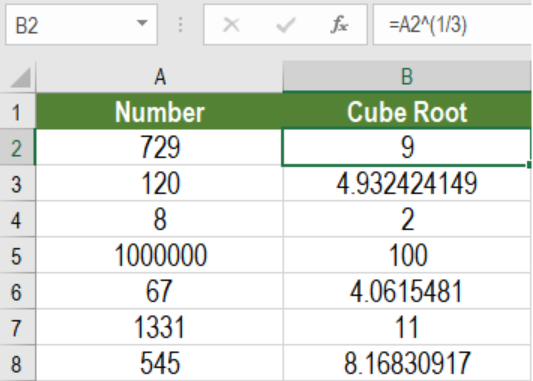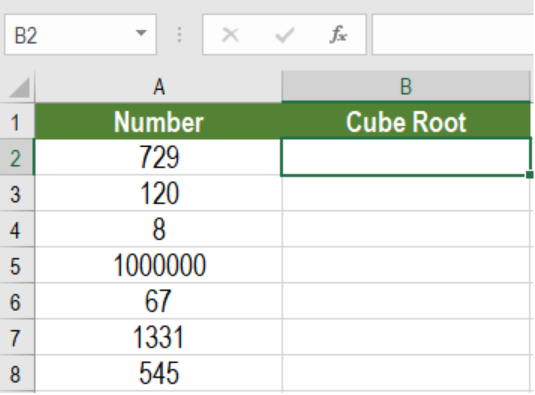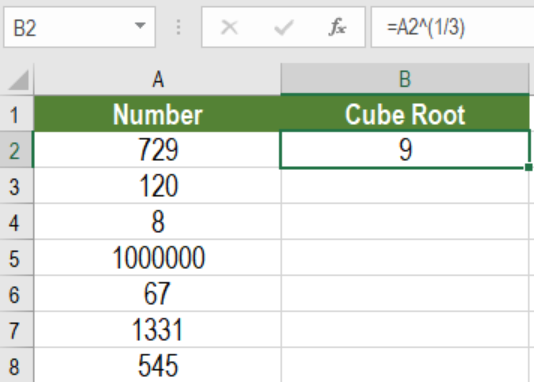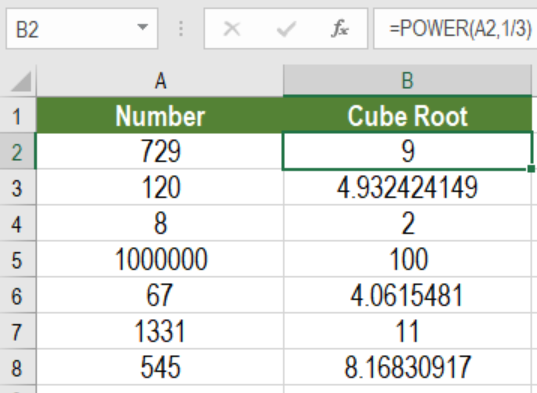Get instant live expert help with Excel or Google Sheets“My Excelchat expert helped me in less than 20 minutes, saving me what would have been 5 hours of work!”

#### Post your problem and you’ll get Expert help in seconds.

Your message must be at least 40 characters
Our professional Expert are available now. Your privacy is guaranteed.

Excel offers many tips and tricks to help its users. One of them is to create a hyperlink to the first match. We can use the HYPERLINK, CELL, INDEX and MATCH functions to create a hyperlink to the first match. It extracts the first match in a lookup and creates the hyperlink for that match. In this article, we will learn how to create a hyperlink to the first match in Excel.Figure 1. Example of How to Hyperlink to the First Match

## Generic Formula

`=HYPERLINK("#"&CELL("address",INDEX(range,MATCH(val,range,0))),val)`

This formula is uses the HYPERLINK function as its core. The other functions used are CELL, INDEX and MATCH. It creates a hyperlink to the first match on the lookup value in the range.

## How this formula works

Here, we use an INDEX and MATCH function to extract the first match of the lookup value in the range. The position of the value found inside the range is extracted by the MATCH function. Excel passes this position value to the INDEX function as row_num argument. The range is provided as the array argument. The INDEX function returns the address of this value. We find this address using the CELL function and concatenate it to the “#” character. Then, we provide this concatenated value to the HYPERLINK function as the link_location. The HYPERLINK function builds a clickable link to the cell address within the same sheet.

## Setting Up Data

The following example uses a fruit sales data set. Column A has the name of the fruits. Column B has the sales.Figure 2. The Fruit Sales Data Set

To create hyperlinks to the first match of the fruits in column E, we need to:

• Go to Cell E9 and click on it with our mouse.
• Assign the formula `=HYPERLINK("#"&CELL("address",INDEX(\$A\$2:\$A\$20,MATCH(D9,\$A\$2:\$A\$20,0))),D9) `in cell E3.
• Next, we need to press Enter to assign the formula to cell E9.Figure 3. Applying the formula to the Data

• Lastly, we need to drag the formula from cells E9 to E12 to copy the formula to the entire column.

This will create clickable links on column E that will redirect to the first match of the fruit names  in column A.

Most of the time, the problem you will need to solve will be more complex than a simple application of a formula or function. If you want to save hours of research and frustration, try our live Excelchat service! Our Excel Experts are available 24/7 to answer any Excel question you may have. We guarantee a connection within 30 seconds and a customized solution within 20 minutes.

### Did this post not answer your question? Get a solution from connecting with the expert.Another blog reader asked this question today on Excelchat:
Solution examplesindex and match with duplicates. I need to use another column as a reference, so my return value has two match the value of two things for it to return
Solved by E. H. in 60 minsHello, I have a big spreadsheet in which I need to know how many patients came in each month based on provider. I am using the following formula but I still get the N/A error. =INDEX('No Show Appts Data'!D:E, MATCH(1, 'No Show Appts Data'!D:D='No Shows Data'!M2)*('No Shows Data'!E:E='No Shows Data'!N1),0)) No Show Appts Data is the name of the sheet where Column D is Month Year of date in question and Column E is the provider. No Shows Data is the sheet where I am making all the formula calculation where Column M is Month and Year and Column N is the provider in question and therefore M2 is the month in question and N1 the provider in question. How do I fix this error? Thanks
Solved by F. H. in 40 minsI need to find an INDEX function that will convert the Call Day (a number 1-7) to the actual weekday found in row 1 of the DayofWeek named range. I don't know if I'm supposed to use the MATCH function as well or not.
Solved by D. D. in 11 minsI have two columns with names and a third one with email addresses. For every name that matches, I need to copy the email address to an empty column next to the matching name. .
Solved by E. U. in 15 minshelp with INDEX(IndexArea,MATCH(\$M\$5,MatchRows,0),MATCH(\$M\$6,MatchColumns,0))
Solved by Z. Y. in 12 mins## Subscribe to Excelchat.coAnother blog reader asked this question today on Excelchat: